# Plus One Maths Chapter Wise Questions and Answers Chapter 4 Principle of Mathematical Induction

Students can Download Chapter 4 Principle of Mathematical Induction Questions and Answers, Plus One Maths Chapter Wise Questions and Answers helps you to revise the complete Kerala State Syllabus and score more marks in your examinations.

## Kerala Plus One Maths Chapter Wise Questions and Answers Chapter 4 Principle of Mathematical Induction

### Plus One Maths Principle of Mathematical Induction Three Mark Questions and Answers

Question 1.
For all n ≥ 1 , prove that
12 + 22 + 32 +……….+ n2 > $$\frac{n^{3}}{3}$$
Let p(n): 12 + 22 + 32 + n2
Put n = 1 ⇒ p(1) = 1 > $$\frac{1}{3}$$ which is true.
Assuming that true for p(k)
p(k): 12 + 22 + 32 +……….+ k2 > $$\frac{k^{3}}{3}$$
Let p(k + 1): 12 + 22 + 32 +…….+ k2 + (k + 1)2 > $$\frac{k^{3}}{3}$$ + (k + 1)2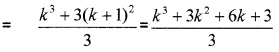Hence by using the principle of mathematical induction true for all n ∈ N.Question 2.
For all n ≥ 1 , prove that 1 + 2 + 3 +…….+ n < $$\frac{1}{8}$$(2n + 1)2
Let p(n): 1 + 2 + 3 +…….+ n ,
Put n = 1 ⇒ p(1) = 1 < $$\frac{9}{8}$$ which is true.
Assuming that true for p(k)
p(k): 1 + 2 + 3 +…….+ k < $$\frac{1}{8}$$(2k + 1)2
Let p(k +1): 1 + 2 + 3 +……..+ k + (k +1) < $$\frac{1}{8}$$ (2k + 1)2 + (k + 1)Hence by using the principle of mathematical induction true for all n ∈ N.

Question 3.
For all n ≥ 1, prove that p(n): 23n – 1 is divisible by 7.
p(1): 23(1) – 1 = 8 – 1 = 7 divisible by 7, hence true. Assuming that for p(k)
p(k) : 23k – 1 is divisible by 7.
23k – 1 = 7M
P(k + 1): 23(k + 1) – 1 = 23k + 3 – 1
= 23k23 – 1 = 23k × 8 – 1
= 23k × 8 – 8 + 7 = 8(23k – 1) + 7
= 8(7M) + 7
Hence divisible by 7. Therefore by using the principle of mathematical induction true for all n ∈ N.Question 4.
For all n ≥ 1, prove that p(n): n3 + (n + 1)3 + (n + 2)3 is divisible by 9.
p(1): 1 + 23 + 33 = 1 + 8 + 27 = 36 divisible by 9, hence true. Assuming that true for p(k)
p(k): k3 + (k + 1)3 + (k + 2)3 is divisible by 9.
⇒ k3 + (k + 1)3 + (k + 2)3 = 9M
p(k +1 ): (k + 1)3 + (k + 2 )3 + (k + 3)3
= (k +1)3 + (k + 2)3 + k3 + 9k2 + 27k + 27
= [(k + 1)3 + (k + 2)3 + k3] + 9[k2 + 3k + 3]
= 9M + 9[k2 + 3k + 3]
Hence p(k + 1)divisible by 9. Therefore by using the principle of mathematical induction true for all n ∈ N.

### Plus One Maths Principle of Mathematical Induction Four Mark Questions and Answers

Question 1.
For all n ≥ 1, prove thatHence by using the principle of mathematical induction true for all n ∈ N.Question 2.
For all n ≥ 1, prove that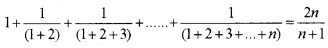LetAssuming that true for p(k)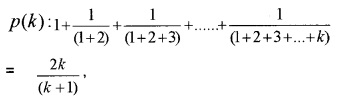Let p(k + 1):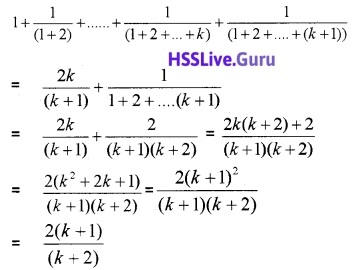Hence by using the principle of mathematical induction true for all n ∈ N.Question 3.
For all n ≥ 1 , prove that 1.2.3 + 2.3.4 +………+ n(n + 1)(n + 2) = $$\frac{n(n+1)(n+2)(n+3)}{4}$$.
Let p(n): 1.2.3 + 2.3.4 +……..+ n(n + 1)(n + 2),
Put n = 1
p(1) = $$\frac{1(1+1)(1+2)(1+3)}{4}$$ = 6 which is true.
Assuming that true for p(k)
p(k): 1.2.3 + 2.3.4 +……..+ k(k + 1)(k + 2) = $$\frac{k(k+1)(k+2)(k+3)}{4}$$,
Let p(k + 1)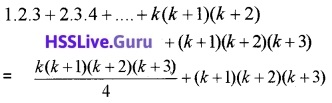Hence by using the principle of mathematical induction true for all n ∈ N.Question 4.
For all n ≥ 1, prove that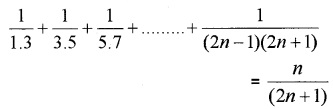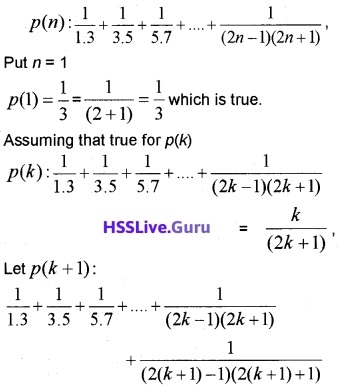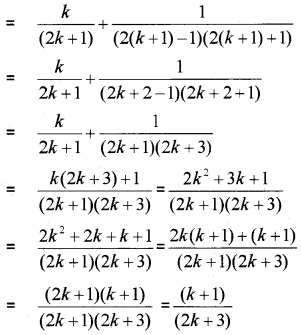Hence by using the principle of mathematical induction true for all n ∈ N.Question 5.
For all n ≥ 1 , prove that p(n): n(n + 1 )(n + 5) is divisible by 3.
p(1): 1(1 + 1)(1 + 5) = 12divisible by 3, hence true. Assuming that true for p(k)
p(k): k(k + 1)(k + 5) is divisible by 3.
k(k + 1)(k + 5) = 3M
p(k + 1): (k + 1)(k + 2)(k + 6)
= (k + 1)(k2 + 8k + 12)
= (k + 1)(k2 + 5k + 3k +12)
= (k + 1)[k(k + 5) + 3(k + 6)]
= [k(k + 1)(k + 5) + 3(k + 1)(k + 6)]
= [3M + 3(k + 1)(k + 6)]
= 3[M + (k + 1)(k + 6)]
Hence divisible by 3. Therefore by using the principle of mathematical induction true for all n ∈ N.Question 6.
For all n ≥ 1 , prove that p(n): 2.7n + 3.5n – 5 is divisible by 24.